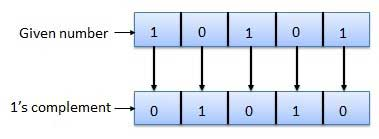# Complement Arithmetic

Complements are used in the digital computers in order to simplify the subtraction operation and for the logical manipulations. For each radix-r system (radix r represents base of number system) there are two types of complements.

S.N. Complement Description
1 Radix Complement The radix complement is referred to as the r's complement
2 Diminished Radix Complement The diminished radix complement is referred to as the (r-1)'s complement

## Binary system complements

As the binary system has base r = 2. So the two types of complements for the binary system are 2's complement and 1's complement.

### 1's complement

The 1's complement of a number is found by changing all 1's to 0's and all 0's to 1's. This is called as taking complement or 1's complement. Example of 1's Complement is as follows.### 2's complement

The 2's complement of binary number is obtained by adding 1 to the Least Significant Bit (LSB) of 1's complement of the number.

2's complement = 1's complement + 1

Example of 2's Complement is as follows.